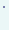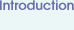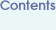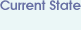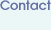# Flow through a Venturi pipe at medium up to high Reynolds number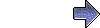Computational details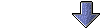Short description and remarksAim of these simulationsQuantitative comparisonsConclusionVisualization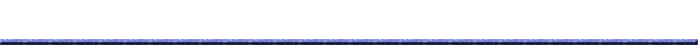Short description and remarks The objective of the following test calculations is to examine in a quantitative and qualitative manner the resulting accuracy of the employed flow solver if it is applied to nonsteady flows with complex behaviour. In contrast to the `flow around a cylinder' Benchmark-simulations which "only" showed periodical vortex shedding behind a circle, we present now results for a flow which exhibits much more complex flow patterns in space and time. The interesting flow quantity in this simulation is the flux through the upper small channel (see the following typical coarse mesh for a sketch of the geometry).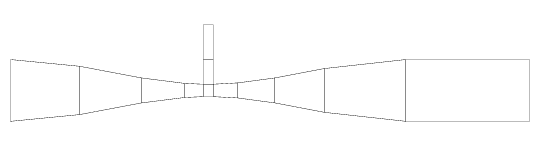In "real life", this Venturi pipe represents a small device used to drain sailing boats. If the flow speed is sufficiently high, then -- due to the Bernoulli principle -- the narrowing section enforces a low pressure which creates a flux through this small device, out of the boat. Therefore, we are mainly interested in controlling the flux through this device, as a function of time. In this simulation (until T=30), the inflow is steady parabolic, and we start from the corresponding Stokes solution. More explanations and remarks can be found in our Paper Archive , particularly in http://www.featflow.de/ture/paper/habil.ps.gz and in Stefan Turek's CFD-book, Springer.Aim of these simulations We aim to show how hard it is - for this medium Reynolds number range - to calculate the `grid independent' solution by simply refining the mesh only, if we ask for a quantitatively exact representation of the flux (through the upper inlet) or the integral mean value of the pressure (on the lower wall)! We employed the following two types of meshes `a' and `b' which are shown for level 3, i.e., after two regular refinements. Grid a:Grid b: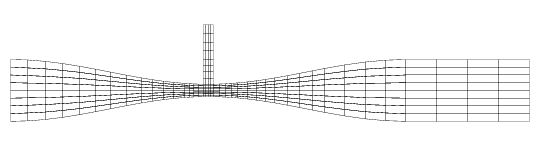Quantitative comparisons The following diagrams show the resulting flux and integral pressure coefficients in time. As explained more carefully in the Computational details , we prescribed a fixed velocity profile only at the left edge, and let the others as free as possible ('zero mean pressure'!). The resulting flux through the upper small device depends on the geometry and Reynolds number; and in this case, the result is that `flow is sucked from the boat'!!! We applied an adaptive time step control with very small error tolerances so that the shown results are (more or less) `exact in time', that means that only the spatial error should be visible! For more information about different types of time stepping techniques (what happens for time steps too large, which time step size is needed to guarantee sufficient accuracy, how does the time step depend on the used `Navier-Stokes solver???) and the comparison of various `Navier-Stokes solvers' (projection-like, fractional step, pressure correction, fully coupled, linearization techniques for the nonlinearity, ...) can be found in the previously described Paper Archive, and particularly in Stefan Turek's CFD-book, Springer. The following plots show the results on different mesh levels (up to level 9!), on meshes `a' and `b'. While the results on levels 8 and 9 show at least some similar dynamic bahviour, we cannot talk about the "grid independent" solution.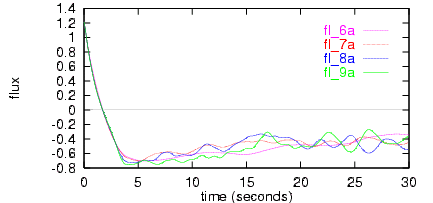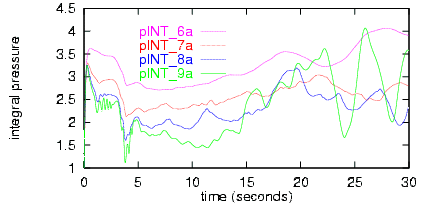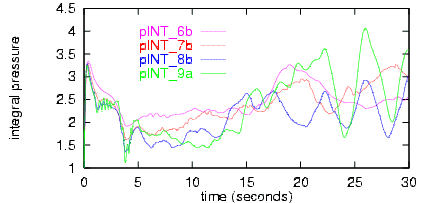Conclusion The following conclusions for flow simulations in this Reynolds number regime can be stated which should be compared with the analogous results for the lower Reynolds number simulations: For this highly nonsteady flow without simple periodical flow patterns a precise numerical simulation seems to be (almost) impossible, at least with the employed simulation and analysis tools if a grid-independent structure shall be obtained. Nevertheless, a qualitative simulation of the dynamic behaviour of the complete flow structure in space and time seems to be possible, at least if we trust the "graphical comparisons" from the following videos. However, the corresponding `exact' prediction of certain flow quantities in a pointwise sense may fail! For corresponding 3D calculations, or for even higher Reynolds numbers, a reduction of mesh points and time steps while maintaining the same accuracy and same efficiency of the solvers is an absolutely necessary task!!! Possible techniques to achieve these improvements are: `Optimal' meshes and `large' time steps (by hand or via error control, second order time stepping) `Better' discretizations of the convective term (by adaptive upwinding or streamline-diffusion techniques) `Faster' and `more robust' solvers (multigrid, better adapted to hardware) `Improved' Navier-Stokes schemes (MPSC techniques) However, the question remains - at least at the moment - whether it is possible at all to calculate the "grid independent" solution for such kind of complex flows, at least with the recently available tools? For the mathematical background concerning these observations and suggestions for improvements, and with respect to general CFD for incompressible flow problems, look at our Paper Archive, and there especially at http://www.featflow.de/ture/paper/habil.ps.gz and in Stefan Turek's CFD-book, Springer.Visualization Distribution of temperature/concentration via Boussinesq model Visualization via tracking of concentration/temperature, starting from the left inflow (size 1) and the upper small device (size -1). We performed the visualization for two different color maps: the first with `red for size 1' and `blue for size -1', while the second one uses `red for absolute size 1' while here blue stands for `blue for size 0' (where both are mixed!). The following rows are for level 9 and 8 - which look very similar at least in a qualitative comparison if both are played at the same time - followed by levels 7 and 6, all calculated on grid `a'. All movies hoccupy about 1 - 2 MB at the most. Pressure Corresponding visualization via shaded pressure plots (less than 1.5 MB). First row for level 9 and 8, second row for level 7 and 6, all on grid `a'. Streamfunction Corresponding visualization via shaded streamline plots (less than 1.5 MB). First row for level 9 and 8, second row for level 7 and 6, all on grid `a'. Velocity Some representative vector plots on level 9 at T=30 (between 8 and 12 MB). The second row shows two zoomed regions.Please send any comments and suggestions to: featflow@featflow.de